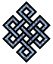#fail2ban bad ip database: ip 116.236.231.246

### | ip database | live view | stats | report | help | api key:

 ip: 116.236.231.246 hostname: 116.236.231.246 country:[CN] China first reported: 25.10.2018 22:05.44 GMT+0200 last reported: 24.03.2019 22:44.27 GMT+0200 time period: 150d 01h 38m 43s total reports: 3 reported by: 2 host(s) filter(s): ssh (2) ssh (1) tor exit node no badips.com db Lookup## port scan of '116.236.231.246':

[-hide]
```# Nmap 6.40 scan initiated Thu Oct 25 22:06:02 2018 as: /usr/bin/nmap -sU -sS -O 116.236.231.246
Nmap scan report for 116.236.231.246
Host is up (0.21s latency).
Not shown: 1000 open|filtered ports, 995 filtered ports
PORT     STATE  SERVICE
22/tcp   closed ssh
80/tcp   open   http
81/tcp   closed hosts2-ns
3389/tcp open   ms-wbt-server
8080/tcp closed http-proxy
No exact OS matches for host (If you know what OS is running on it, see http://nmap.org/submit/ ).
TCP/IP fingerprint:
OS:SCAN(V=6.40%E=4%D=10/25%OT=80%CT=22%CU=%PV=N%G=Y%TM=5BD2226E%P=x86_64-pc
OS:-linux-gnu)SEQ(SP=103%GCD=1%ISR=10E%TI=I%TS=7)OPS(O1=M5A8NW8ST11%O2=M5A8
OS:NW8ST11%O3=M5A8NW8NNT11%O4=M5A8NW8ST11%O5=M5A8NW8ST11%O6=M5A8ST11)WIN(W1
OS:=2000%W2=2000%W3=2000%W4=2000%W5=2000%W6=2000)ECN(R=Y%DF=Y%TG=80%W=2000%
OS:O=M5A8NW8NNS%CC=N%Q=)T1(R=Y%DF=Y%TG=80%S=O%A=S+%F=AS%RD=0%Q=)T2(R=N)T3(R
OS:=N)T4(R=N)T5(R=Y%DF=Y%TG=80%W=0%S=Z%A=S+%F=AR%O=%RD=0%Q=)T6(R=N)T7(R=N)U
OS:1(R=N)IE(R=N)

OS detection performed. Please report any incorrect results at http://nmap.org/submit/ .
# Nmap done at Thu Oct 25 22:07:10 2018 -- 1 IP address (1 host up) scanned in 68.61 seconds
```
```Σ = 80 | Δt = 0.0046970844268799s
```